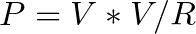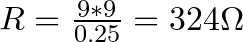# How To Choose A Pull-up Resistor Value

I recently got a question about choosing a pull-up resistor value:

Hey!
In Monostable button triggered circuit, why did you connect 10 kΩ specifically? Does it contain any calculations?

You can use resistors for many things. In the following 555 timer circuit that he was referring to, the resistor R2 is a pull-up resistor with a value of 10 kΩ:

The 10k resistor (R2) is there the make sure the voltage on the pin is held at a stable high level.

“High” as in a value close to the supply voltage.

The reason that I chose the value 10k is that from my experience I know that 10k to 100k pull-up resistors usually work fine in almost all circuits.

I’ve seen this used many times and I’ve tried it myself many times.

But if you don’t know this and you need to find a value, read on.

## Choosing a Pull-up Resistor Value

There are two basic guidelines for choosing a pull-up resistor value.

You need a value that is:

1. Not too small
2. Not too big

### Not Too Small

The first factor is to have a value that is not too small.

If the value is too small a lot of current will flow through the resistor, which makes it really warm and could break it.

What is “too small”?

Standard resistors often have a power rating of 0.25W (Watt).

If you look at a worst-case scenario, all the 9V of the voltage source would drop across the resistor.

Power dissipation in a resistor can be calculated with this formula:We can solve that for R to find the minimum resistance value you need:With a resistor of 324 Ω you’ll be at the limit of what the resistor can handle if there is a 9V drop over it.

So you want to have a value much higher than this.

If you choose a value of minimum 10-20 times this, you should be fine. For example 5 kΩ as a minimum.

### Not too big

The resistor value cannot be too big.

If the resistor value is too big, you get too much voltage drop over it so that the value on the input pin is no longer high enough to be called high.

What is “too big”?

You can use this rule of thumb:

Choose a pull-up resistor value that is at least 10 times smaller than the input impedance of the pin.

(This is because the input impedance and the pull-up resistor will make up a voltage divider. Keeping it 10 times lower assures the drop across the resistor is less than 10%.)

The input impedance of the 555 timer is 10 Megaohms according to electronicsclub.info. And 10 times smaller is 1 MΩ.

That gives you the choice of a pull-up resistor value of anywhere in between 5 kΩ and 1 MΩ.

So as you can see, you can use a range of values here.

## Do You Have Any Questions?

Do you have any questions about choosing pull-up resistor values? Let me know in the comment field below.

## More Resistors Tutorials

### 6 thoughts on “How To Choose A Pull-up Resistor Value”

1. thanks so much

2. How to distinguish between pull-up resistor and normal resistor ???